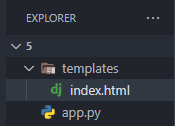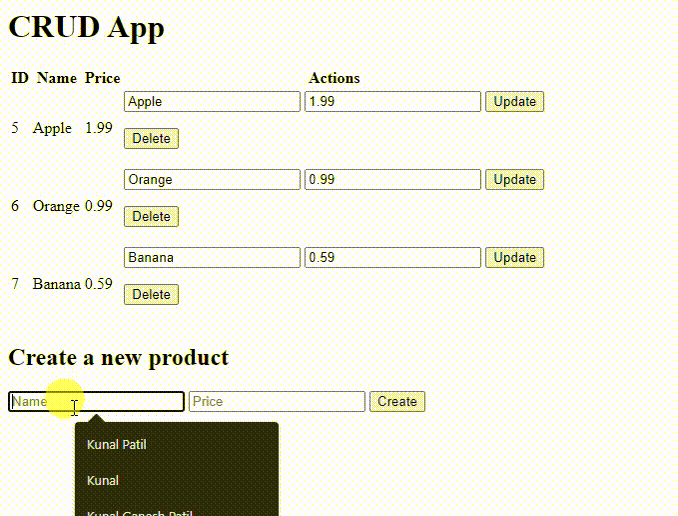GeeksforGeeks App
Open AppBrowser
Continue

# Making a Flask app using a PostgreSQL database

The Postgres database can be accessed via one of two methods in Python. Installing PgAdmin4 is the first step because it offers a user interface for interacting with databases and another for using the psycopg2 connector. In this post, we’ll concentrate on a different approach that lets us alter the Postgres database: using the psycopg2 connector. This article presupposes that Postgres has been successfully installed on your computer or device.

Let’s get started on the project. An HTML page will be added to display the modifications, along with a few products and their prices. It will include the three tables. earliest for product details. second to update existing items, and third to add new ones.

### Steps to make Flask app using a PostgreSQL Database

Step 1: Open your command line tool and connect with Postgres. After entering the username it will ask you for the password enter it appropriately.

`psql -U username`

Step 2: Once we successfully connected then create a new database ‘flask_db’. We will use it further to create tables in it.Step 3: Create a folder structure like this.Step 4:  Connect with the database. you will have to use psycopg2 connect to use the commands.

## Python3

 `conn ``=` `psycopg2.connect(database``=``"flask_db"``, ``                        ``user``=``"postgres"``,``                        ``password``=``"root"``, ``                        ``host``=``"localhost"``, port``=``"5432"``)`` ` `cur ``=` `conn.cursor()`` ` `conn.commit()`` ` `cur.close()``conn.close()`

Step 5:  app.py

This will authenticate the user and allows us to execute queries on it. It creates a cursor on the connection by using cur. execute() method executes the queries for us. Once we completed our queries we have to close the connection for security purposes.

## Python3

 `from` `flask ``import` `Flask, render_template, request, redirect, url_for``import` `psycopg2`` ` `app ``=` `Flask(__name__)`` ` `# Connect to the database``conn ``=` `psycopg2.connect(database``=``"flask_db"``, user``=``"postgres"``,``                        ``password``=``"root"``, host``=``"localhost"``, port``=``"5432"``)`` ` `# create a cursor``cur ``=` `conn.cursor()`` ` `# if you already have any table or not id doesnt matter this ``# will create a products table for you.``cur.execute(``    ``'''CREATE TABLE IF NOT EXISTS products (id serial \``    ``PRIMARY KEY, name varchar(100), price float);'''``)`` ` `# Insert some data into the table``cur.execute(``    ``'''INSERT INTO products (name, price) VALUES \``    ``('Apple', 1.99), ('Orange', 0.99), ('Banana', 0.59);'''``)`` ` `# commit the changes``conn.commit()`` ` `# close the cursor and connection``cur.close()``conn.close()`` ` ` ` `@app``.route(``'/'``)``def` `index():``    ``# Connect to the database``    ``conn ``=` `psycopg2.connect(database``=``"flask_db"``,``                            ``user``=``"postgres"``,``                            ``password``=``"root"``,``                            ``host``=``"localhost"``, port``=``"5432"``)`` ` `    ``# create a cursor``    ``cur ``=` `conn.cursor()`` ` `    ``# Select all products from the table``    ``cur.execute(``'''SELECT * FROM products'''``)`` ` `    ``# Fetch the data``    ``data ``=` `cur.fetchall()`` ` `    ``# close the cursor and connection``    ``cur.close()``    ``conn.close()`` ` `    ``return` `render_template(``'index.html'``, data``=``data)`` ` ` ` `@app``.route(``'/create'``, methods``=``[``'POST'``])``def` `create():``    ``conn ``=` `psycopg2.connect(database``=``"flask_db"``,``                            ``user``=``"postgres"``,``                            ``password``=``"root"``,``                            ``host``=``"localhost"``, port``=``"5432"``)`` ` `    ``cur ``=` `conn.cursor()`` ` `    ``# Get the data from the form``    ``name ``=` `request.form[``'name'``]``    ``price ``=` `request.form[``'price'``]`` ` `    ``# Insert the data into the table``    ``cur.execute(``        ``'''INSERT INTO products \``        ``(name, price) VALUES (%s, %s)'''``,``        ``(name, price))`` ` `    ``# commit the changes``    ``conn.commit()`` ` `    ``# close the cursor and connection``    ``cur.close()``    ``conn.close()`` ` `    ``return` `redirect(url_for(``'index'``))`` ` ` ` `@app``.route(``'/update'``, methods``=``[``'POST'``])``def` `update():``    ``conn ``=` `psycopg2.connect(database``=``"flask_db"``,``                            ``user``=``"postgres"``,``                            ``password``=``"root"``,``                            ``host``=``"localhost"``, port``=``"5432"``)`` ` `    ``cur ``=` `conn.cursor()`` ` `    ``# Get the data from the form``    ``name ``=` `request.form[``'name'``]``    ``price ``=` `request.form[``'price'``]``    ``id` `=` `request.form[``'id'``]`` ` `    ``# Update the data in the table``    ``cur.execute(``        ``'''UPDATE products SET name=%s,\``        ``price=%s WHERE id=%s'''``, (name, price, ``id``))`` ` `    ``# commit the changes``    ``conn.commit()``    ``return` `redirect(url_for(``'index'``))`` ` ` ` `@app``.route(``'/delete'``, methods``=``[``'POST'``])``def` `delete():``    ``conn ``=` `psycopg2.connect``    ``(database``=``"flask_db"``, user``=``"postgres"``,``     ``password``=``"root"``,``     ``host``=``"localhost"``, port``=``"5432"``)``    ``cur ``=` `conn.cursor()`` ` `    ``# Get the data from the form``    ``id` `=` `request.form[``'id'``]`` ` `    ``# Delete the data from the table``    ``cur.execute(``'''DELETE FROM products WHERE id=%s'''``, (``id``,))`` ` `    ``# commit the changes``    ``conn.commit()`` ` `    ``# close the cursor and connection``    ``cur.close()``    ``conn.close()`` ` `    ``return` `redirect(url_for(``'index'``))`` ` ` ` `if` `__name__ ``=``=` `'__main__'``:``    ``app.run(debug``=``True``)`

Step 4: templates/index.html

You will need this index file to see results and perform operations. Just add these template codes to their respective path.

## HTML

 `<``html``>``  ``<``head``>``    ``<``title``>CRUD App``  ````  ``<``body``>``    ``<``h1``>CRUD App``    ``<``table``>``      ``<``thead``>``        ``<``tr``>``          ``<``th``>ID``          ``<``th``>Name``          ``<``th``>Price``          ``<``th``>Actions``        ````      ````      ``<``tbody``>``        ``{% for row in data %}``        ``<``tr``>``          ``<``td``>{{ row }}``          ``<``td``>{{ row }}``          ``<``td``>{{ row }}``          ``<``td``>``            ``<``form` `action``=``"/update"` `method``=``"post"``>``              ``<``input` `type``=``"hidden"` `name``=``"id"` `value``=``"{{ row }}"``>``              ``<``input` `type``=``"text"` `name``=``"name"` `value``=``"{{ row }}"``>``              ``<``input` `type``=``"text"` `name``=``"price"` `value``=``"{{ row }}"``>``              ``<``input` `type``=``"submit"` `value``=``"Update"``>``            ````            ``<``form` `action``=``"/delete"` `method``=``"post"``>``              ``<``input` `type``=``"hidden"` `name``=``"id"` `value``=``"{{ row }}"``>``              ``<``input` `type``=``"submit"` `value``=``"Delete"``>``            ````          ````        ````        ``{% endfor %}``      ````    ````    ``<``h2``>Create a new product``    ``<``form` `action``=``"/create"` `method``=``"post"``>``      ``<``input` `type``=``"text"` `name``=``"name"` `placeholder``=``"Name"``>``      ``<``input` `type``=``"text"` `name``=``"price"` `placeholder``=``"Price"``>``      ``<``input` `type``=``"submit"` `value``=``"Create"``>``    ````  `````

Step 4: Run our app

Enter the flask run command to run it in the development mode and make sure the flask debug is on.

`flask run`

Through this, we have successfully executed Crud operations on the database. You will have the output attached to this and a GitHub repository link where you can find all the source code.

Output:My Personal Notes arrow_drop_up